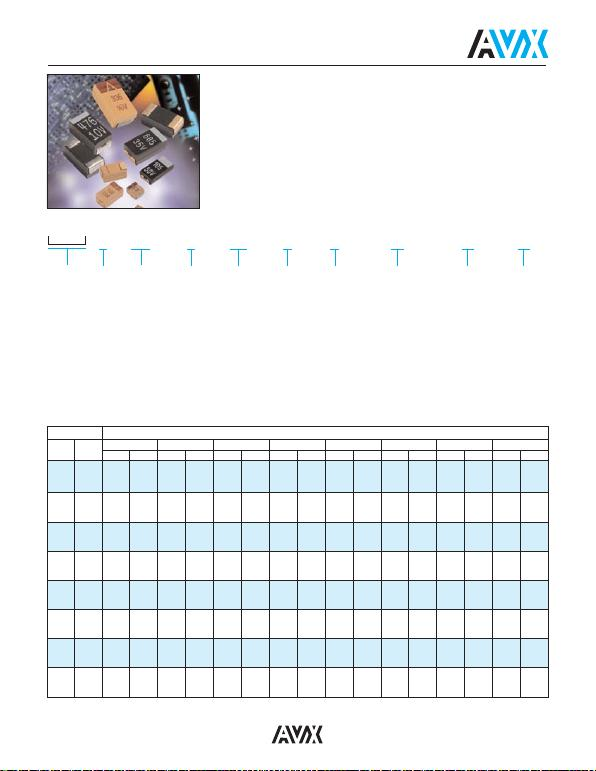19
SRC9000 Series
High Reliability Tantalum Capacitors for Space Applications
AVX SRC9000 microminiature capacitors are designed and built to meet the high
reliability and long term requirements of military space applications. All SRC9000
capacitors meet all of the requirements of Mil-PRF-55365 and include DPA
requirements per MIL-STD-1580. SRC9000 establishes a rigorous screening test
schedule designed to detect and eliminate from shipment any capacitor or capacitor
test lots that exhibits poor performance or reliability. SRC9000 establishes a
continuous test schedule to determine baseline reliability data for specific product
shipped under this specification. SRC9000 assures that proper lot control and lot
traceability procedures are in effect.
Type
(3 letters)
TBJ
TAZ
HOW TO ORDER
90
Termination
Finish
90 = SRC9000
#@
Qualification/
Reliability
# = Inspection Level
S = Std. Conformance
L = Group A
@ = Failure Rate Level
Weibull:
B = 0.1%/1000 hrs,
90% conf.
C = 0.01%/1000 hrs,
90% conf.
D = 0.001%/1000 hrs,
90% conf.
Comm: Z = Non ER
227
Capacitance
Code
006
Voltage
Code
004 = 4Vdc
006 = 6Vdc
010 = 10Vdc
015 = 15Vdc
020 = 20Vdc
025 = 25Vdc
035 = 35Vdc
050 = 50Vdc
*
Capacitance
Tolerance
M = ±20%
K = ±10%
J = ±5%
D
Case
Size
Packaging
B = Bulk
R = 7" T&R
S = 13" T&R
++
Surge Test
Option
00 = None
23 = 10 cycles,
+25°C
24 = 10 cycles,
-55°C &
+85°C
45 = 10 cycles,
-55ºC &
+85ºC
before
Weibull
C
Standard or
Low ESR
Range
C = Std ESR
L = Low ESR
Capacitance Voltage Rating DC (VR) to 85°C
4V 6V 10V 15V 20V 25V 35V 50V
µF Code TAZ TBJ TAZ TBJ TAZ TBJ TAZ TBJ TAZ TBJ TAZ TBJ TAZ TBJ TAZ TBJ
0.1 104 A A A
0.15 154 AAA
(M)
/B
0.22 224 AABA
(M)
/B
0.33 334 AAAABB
0.47 474 A A A A B A
(M)
/B C C
0.68 684 A A A/B A B A/B C A
(M)
/B D C
1 105 A A A A A/B A B/C A/B D A/B E C
1.5 155 A A A A/B A B/C A/B D A/B E A/B/C F C/D
2.2 225 A A A A/B A A/C A/B B/D B D/E A/B/C B/C F D
3.3 335 A A/B A A/C A/B B/D A/B D/E B E B/C F B/C G D
4.7 475 A/B A A/C A/B B/C/D A/B B/C/D/E A/B E A/B/C F B/C G B/C/D H D
6.8 685 A/C A/B B/D A/B B/C/D/E A/B D/E A/B/C E/F B/C F/G B/C/D G/H C/D D
10 106 B/D A/B B/E A/B B/C/D/E A/B/C D/E/F B/C E/F B/C G C/D H C/D
15 156 B/E A/B B/D/E A/B/C D/E/F A/B/C E/F B/C F/G B/C/D G/H D X C/D
22 226 B/D A D/E/F A/B/C E B/C F/G B/C/D G/H C/D G/H/X C/D D/E
33 336 D/E/F A/B/C E B/C F/G B/C/D F/G/H C/D H C/D H/X D/E D
(M)
47 476 E B F/G C/D F/G/H C/D G/H C/D H/X D D
(M)
68 686 E/G C/D F/G/H B/C/D G C/D G/H D D/E V
100 107 F/H B/C/D G C/D G/H C/D H D/E V
150 157 G G D H/X D D
(M)
/V
220 227 H D H C/D H D
(M)
/E V
330 337 H E H E D
(M)
/E
470 477 H E
(M)
/V E
(M)
/V
680 687
CAPACITANCE AND RATED VOLTAGE, VR(VOLTAGE CODE) RANGE
(LETTER DENOTES CASE SIZE)